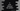# C program to print a diamond pattern using star or any characterIn this tutorial, we will learn how to print the diamond star pattern using C programming language. User will give the input for number of rows of the star pattern and we will print out the result. For rows count 5, it will print like below :

``````    *
***
*****
*******
*********
*******
*****
***
*``````

### How to print a pattern like this ?

To understand this pattern, let’s replace the space with \$ :

``````\$\$\$\$*
\$\$\$***
\$\$*****
\$*******
*********
\$*******
\$\$*****
\$\$\$***
\$\$\$\$*``````
1. So, for the first line, we will print 4 ’\$” or space _ and one _’*’ . For the second line, 3 ’\$’ and 3 ’*’ etc.
2. For the first 5 lines, on each line, ’\$’ is decrementing by one, starting from row count - 1. And, ’*’ for each line is 2 * (row index - 1). Row index is starting from 1. 3. For the last 4 lines, on each line, ’\$’ is printed for 1,2,3,4… times.
3. The value of ’*’ is printed for 2 * (row count - i) - 1 times.

We will divide the program into two parts. First part will print the first half of the triangle and the second half will print the second half of the triangle. Let’s take a look into the program first :

### C program :

``````#include

int main()
{
//1
int i,j,row,space;

//2
printf("Enter total number of rows : \n");
scanf("%d", &row);

//3
space = row - 1;

//4
for (i = 1; i <= row; i++)
{
for (j = 1; j <= space; j++)
printf(" ");

space--;

for (j = 1; j <= 2*i-1; j++)
printf("*");

printf("\n");
}

//5
space = 1;

//6
for (i = 1; i <= row - 1; i++)
{
for (j = 1; j <= space; j++)
printf(" ");

space++;

for (j = 1 ; j <= 2*(row-i)-1; j++)
printf("*");

printf("\n");
}

return 0;
}``````

### Explanation :

The commented numbers in the above program denotes the step number below :

1. First create four integer variables i,j,row and space
2. Ask the user to enter the rows number and store it in row variable.
3. Initialize the space variable as row -1 . We will print blank space as this variable number of times.
4. This for loop will print the first half of the triangle. If the row count is 5, it will print first 5 lines of the triangle.
5. After the loop is completed, reassign space to 1.
6. This for loop will print the below half of the triangle. The final triangle will look as above.

### Example :

``````       *
***
*****
*******
*********
***********
*************
***************
*************
***********
*********
*******
*****
***
*

*
***
*****
*******
*********
***********
*************
***************
*****************
*******************
*****************
***************
*************
***********
*********
*******
*****
***
*``````